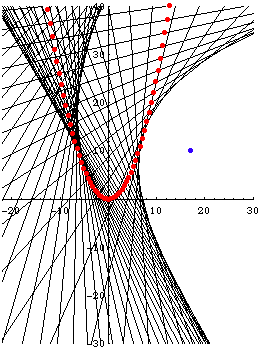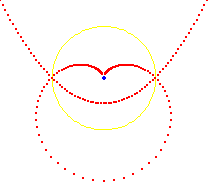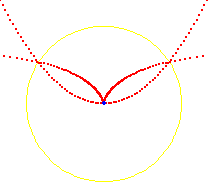ParabolaTwo negative pedals of the parabola {t,1/4 t^2}

Description

Parabola is a member of conic sections, along with hyperbola and ellipse. Parabola can be thought of as a limiting case of ellipse or hyperbola. Note that parabola is not a family of curves. The impression that some parabola are more curved is because we are looking at different scale of the curve. Similarly, part of a large circle appears to be a line may induce us to conclude that there are different shapes of circles.

Like ellipse and hyperbola, there are many ways to define parabola. A common definition defines it as the locus of points P such that the distance from a line (called the directrix) to P is equal to the distance from P to a fixed point F (called the focus). As a conic section, the eccentricity of Parabola is 1.

The axis of a parabola is a line perpendicular to its directrix and passing its focus. Vertex of the parabola is the intersection of the parabola and its axis.

Formula

• Parametric: {t, 1/4 t^2}, -∞ < t < ∞
• Cartesian: y == 1/4 x^2.

For the given formulas, vertexes is at {0,0}, focus is at {0,1}.

Properties

Point and Tangent Construction

Let F be a given point and d be a give line. Let B := Point[d]. Let t := LineBisector[B,F]. Let b := Perpendicular[B,d]. Let P := Intersect[b,t] Since length[segment[B,P]]==length[segment[P,F]], P is a point on parabola. Further, t is the tangent at P.

Invariant under certain Dilation

Parabola have the property that when scaled (streching/shrinking) along a direction parallel or perpendicular to its axis, the curve remain unchanged. (For example, line also have this property, but circle do not. A streched line is still a line, but a streched circle is no longer a circle) When a parabola is streched along the directrix “a” units and along the axis by “b” units, the resulting curve is the original parabola scaled in both direction by “a^2/b”.

Given a parametrization of a parabola {xf[t], yf[t]} with vertex at Origin and focus along the y-axis, its focus is {0, xf[t]^2/(4 yf[t]) }.

Optical Property

A radiant point at the focus will reflect off the parabola into parallel lines. The figure shows three parabolas, two of which share a common focus.

Tangents of Probala

Any set of tangents on the parabola will always cut a arbitrary tangent into the same proportion. That is, suppose you pick three tangents call them a, b, c. Now pick a arbitrary tangent x. Tagents a, b, c will cut x into segments with certain proportions. Now pick any other tangent x1, it will be cut into the same proportions. Thus, the envelope of lines with a positive constant sum of intercepts is a segment of parabola.Tangents of parabala cutting other tangents into the same proportion.A segment of parabola formed by envelope of lines. The left figure shows the line positions, the right figure is rotated to visually show that it coincides with parabola in the standard position. Note: this is not astroid, because the lines that forms astroid as Trammel of Archimedes do not have the same positions as this.

Evolute and Semicubic Parabola

The evolute of a parabola is the semicubic parabola.Parabola and its normals. The envelope of the normals is the semicubic parabola.

PedalThe pedal of a parabola with respect to its focus is a linepedal with respect to its vertex is the cissoid of Diocles.

InversionThe inversion of a parabola with respect to its focus is a cardioidinversion with respect to its vertex is the cissoid of Diocles

Misc

A fun animation of string art, based on the idea of parabola by envelope: string_art_square.mov string_art_movie.nb.zip

Related Web Sites

Robert Yates: Curves and Their Properties.

If you have a question, put \$5 at patreon and message me.